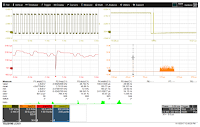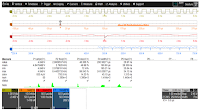## You need to test, we're here to help.

You need to test, we're here to help.

## 31 January 2018

### Getting The Most Out Of Your Oscilloscope: Tracks and TrendsFigure 1: The track math function shows how data changes over time
Our discussion of cursors and parameters leads us neatly into the topics of tracks and trends, which are both means of extending parameter measurement into a more analytical direction. We can do this using math functions that are part of your Teledyne LeCroy oscilloscope's toolset.

As your oscilloscope acquires waveforms and automatically compiles statistical data on parameter measurements, the track math function gives you a way to understand how a given parameter might (or might not) be changing over time.

Setting up a track math function entails touching a parameter measurement (such as P4, pulse width, in Figure 1) and selecting Track from the shortcut options. A track, in essence, is a waveform of the measurement values. The parameter measurement values are resampled so that the track waveform is time-coherent with the source waveform. The track function of the pulse-width parameter measurement appears at center left in Figure 1. At top right is a zoomed trace showing a glitch in the waveform. That glitch appears time-correlated as the large dip in pulse width at the center of the track function trace.Figure 2: A screen capture showsexamples of a histogram (F5, orange),a track (F6, red), and a trend (F7, blue)
The track math function can be used in many different ways. It can show us how power-supply ripple or droop changes over time, or how jitter or time-interval error changes over time. The function's power stems from the fact that all instances of the measurement are displayed and accounted for. Then, you can use cursors to determine precise values.

Trend functions are taken from the same parameter measurement data as the track function, but rather than being time-correlated with the source waveform, the points in the trend function are simply plotted by the order in which the measurements were made. The trend function is typically applied as a data logger showing the history of measured parameter values. If trends of two or more parameters are created simultaneously, they can be plotted on an X-Y display to investigate functional relationships.Figure 3: Parameter qualificationindicators tell us whether a givenparameter was measured correctly
Figure 2 depicts a histogram (F5, orange), a track function (F6, red), and a trend function (F7, blue) of P4, the pulse-width measurement of our glitchy waveform from Figure 1. As in Figure 1, we can zero in on the glitch in time-correlated fashion with the track function. But from the trend function, we note that our glitch is periodic in nature vs. random.

A final note on the parameter table concerns the parameter qualification indicators that can be seen at bottom of the table in the row labeled "status." An example appears in Figure 3. These indicators help us determine whether a given parameter measurement is being taken correctly. The rise-time measurement P1 displays no value simply because the displayed waveform doesn't have a rising edge. The fall-time measurement P2 shows a caution symbol due to a paucity of measurements. Only the amplitude measurement P3 is valid.

Next time, we'll begin discussing some measurement and analysis tools.

Previous posts in this series:

Getting The Most Out Of Your Oscilloscope: Setup